# GAT Analytical Reasoning Lesson-01Basic GAT Analytical Reasoning Lesson-01

GAT Analytical Reasoning

Before going ahead in this lesson, which is part of complete GAT Preparation Online course, it is extremely important to read the answers of the following frequently asked questions about GAT Analytical Reasoning section:

# Important Frequently Asked Questions regarding GAT Analytical Reasoning:

## What is tested in GAT Analytical Reasoning?

This section grades you on how efficiently you can reason to solve logical puzzle based on given sets of conditions (sometimes called as limitations) in such a short span of time. Sometimes, it is also called group reasoning, where group of information is given and you need to answer based on the given information. If you can accurately extract information in a diagrammatic style, such questions become easier. In this section, your brain’s work of analyzing & diagramming (or sketching) the information is much more critical. This section tests your Analytical Reasoning skill in addition to Time Management.

## What’s the trick to get 99th percentile rank in GAT Analytical Reasoning Section?

Keep the following important points in mind that would help you to achieve the target score in this section:

• Read the group information carefully, and draw sketch accurately. Never miss any information while drawing your sketch, because your sketch will make your answers not only correct but also quick. In other words, making an accurate sketch is the key to succeed in GAT Analytical Reasoning section.
• Once, sketch is done, never go back to the group information so that you can save plenty of time by simply analyzing your sketch. There’s very limited time in your exam. So, if you want to complete analytical section within time, you need lot of good practice which is available in this complete course
• .

• In preparation stage, give more time to understand sketch techniques. Once you become expert in making accurate sketch, you will become expert in GAT Analytical Reasoning.
• Never get panic or feel anxious when it takes plenty of minutes to solve a question in preparation stage, because you need to practice your brain with such new sort of questions. Once, your brain get habit to deal with such questions, you will able to answer quickly. So, be self-motivated!
• Remember, that once you complete the 20-days study plan as mentioned in this online course, GAT Analytical Reasoning section will become in your fingertips. And you can get 99th percentile rank in GAT Analytical Reasoning.

You should know the exact meaning of the following questions:

• Which of the following Must be true?
• From the given choices below, which one Could be true?
• Which of the following Cannot be true?
• All of the following will be true EXCEPT?
• All of the following could be true EXCEPT?
• Each of the following cannot be true EXCEPT?

### Must be true:

It is a situation when the given information is always true or there’s no any possibility when the given information is false. In other words, there’s 100% chance that the information is true, or there’s 0% chance that the given information is false. To understand this, let’s suppose, if X is the father of Y and Z. Then, age of X must be greater than age of Y. Also, age of X must also be greater than age of Z. But age of X is not must be greater than combined ages of Y and Z.

### Could be true:

It is a situation when the given information may possibly be true or there’s a possibility that the information may be false. In other words, we are not certain whether the information is true, but there’s just some possibility or chance that the information can be true. To understand this, let’s suppose the same example, i.e.: if X is the father of Y and Z. Then, age of X could be greater than combined ages of Y and Z. It means that there’s a possibility that age of X can be greater than the sum of the ages of Y and Z. But this information is not must be true. Also, we cannot say that this information cannot be true, because there’s a possibility that the information can be true.

### Cannot be true:

It is a situation where the given information is always false or there’s no any possibility when the given information is true. In other words, there’s 100% chance that the information is false, or there’s 0% chance that the given information is true. Let’s suppose, if X is the father of Y and Z. Then, age of Y cannot be greater than age of X. Also, age of Z cannot also be greater than age of X.

### Will be true EXCEPT:

It means that all of the given choices will always be true, except one choice, which can be false. The choice which can be false is correct answer. Let’s consider, if X is the father of Y and Z, all of the following will be true except the last choice:

A)   Age of X is greater than age of Y
B)   Age of X is greater than age of Z
C)   Combined ages of X and Y is greater than age of Z
D)   Combined ages of X and Z is greater than age of Y
E)   Height of Z is greater than height of X

### Could be true EXCEPT:

It means that all of the given choices can be true, except one choice, which cannot be true. So, the choice which cannot be true is correct. Let’s consider, if X is the father of Y and Z, all of the following can be true except the last choice:

A)   Both Y and Z are sons of X
B)   Both Y and Z are daughters of X
C)   Age of Y is greater than age of Z
D)   Height of Z is greater than height of X
E)   Age of Y is greater than age of X

### Cannot be true EXCEPT:

It means that all of the given choices is false, except one choice, which can be true. So, the choice which can be true is correct. Let’s consider, if X is the father of Y and Z, all of the following cannot be true EXCEPT the last choice:

A)   Age of Y is greater than age of X
B)   Age of Z is greater than age of X
C)   X has no child
D)   X, Y and Z are siblings
E)   X is having least height among X, Y and Z

GAT Analytical Reasoning questions are presented in groups of three to seven questions. Each group is based on a short passage followed by a set of conditions. There are some basic strategies that would help you to solve GAT Analytical Reasoning questions at beginner stage.

## Basic Strategies for GAT Analytical Reasoning:

• Always count the number of questions in a given group and start from the group which contains largest number of questions. It’ll not be good if you give huge time to that group which contains only few questions.
• Utilize maximum one and a half minutes per question on average. i.e., if there are 4 questions in a group, spend no more than 6 minutes to this group. If a question is left despite 6 minutes would over, take a minute to try. Otherwise if more than one questions still left; just move on to next group and avoid further waste of time, because next group may be relatively easier.
• Always draw “intelligent drawing” (i.e., shape the information of the group in picture form or a sketch) of each group while reading the passage containing information. There’s no hard and fast rule for drawing the sketch. Everybody has his own way to solve the questions and create different drawing on the spot.
• Make changes in the drawing according to the additional information that are subject to limitations in the group question. And let’s call it as your original sketch.
• When the drawing finalize according to all information of group, then move on the first question of the group and change the drawing according to the question, if necessary. And answer the first question, while using the drawing.
• While solving the next question, always revert back to the original drawing rather than the drawing used in first question after changes. And similarly, while solving 3rd and 4th questions use the original drawing to find the answer.

Important Note: If you successfully answered the first question from the group having several questions and proceeded to answer the second one, just forget about the information given in the first question; just consult the information in the passage including conditions. Similarly if you answered the second question of the group of several questions and proceeded to the third one, just forget about the information given in first and second questions of the group; again consult the information in the passage of the group together with the conditions..

## Example of Analytical Question:

To understand the general format of the question, consider the following simple example scenario at this beginners stage. In advance level, we’ll discuss actual questions scenarios with medium and hard difficulty levels questions with according strategies. After covering that complete, you will become expert in analytical reasoning section.

### Basic GAT Analytical Reasoning example scenario:

#### Answer Question 1 – 4 based on information below:

Five people (namely: A, B, C, D, and E) took GAT exam, and were got ranked according to the given condition:
– Mr. A was amongst top Three in the exam.
– Mr. B was on Fourth rank.
– C was on the adjacent rank next to E.

1. Which of the following MUST be on the last rank?
a) Mr. A
b) Mr. B
c) Mr. C
d) Mr. D
e) Mr. E

2. Which of the following MUST be on the first rank, if B was on next adjacent rank next to A?
a) Mr. A
b) Mr. B
c) Mr. C
d) Mr. D
e) Mr. E

3. All of the following could be the true ranking of persons from top to bottom EXCEPT?
a) A, E, C, B, D
b) E, C, A, B, D
c) E, A, C, B, D

4. How many possible arrangements of ranking of five persons can be made?
a) 0
b) 1
c) 2
d) 3
e) 4

Looking for the Best Book for GAT (General) Preparation? After 100% success rate of online course, we have published The Best Book for GAT (General) – by Earnest Prep. You can order the book with FREE HOME DELIVERY by clicking Order GAT Book button below:

To answer these questions, always make arrangements according to the conditions given in question. i.e.,

• First condition (or we may say first restriction) tells information about the possible positions of A, that may only be placed on first three positions as shown below: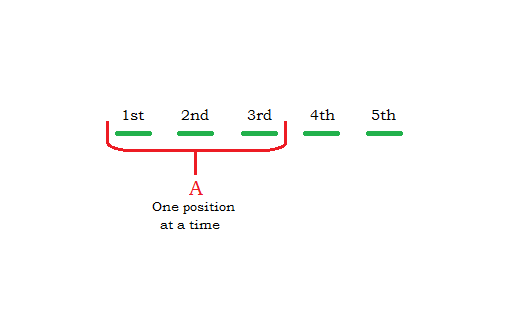• Second condition (or restriction) tells information about the position of B, which must be placed at fourth position/rank. i.e.,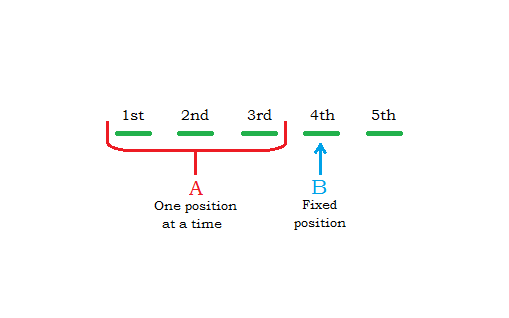• Third and final condition tells information about the places of C and E, that must be place on adjacent places, i.e., place side-by-side; such that C must place after E place. The word “next to” signify that C must place just after the rank of E, i.e., not before E place. Because both have to be place together, so neither C nor D can place at 5th position/rank and, therefore, can be placed at first three positions, as shown below: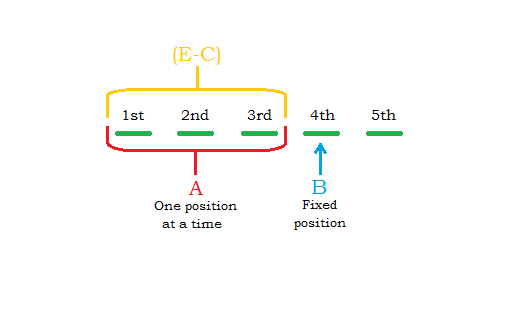Finally, one person D is left with only a position of 5th; So it must be fixed on this position, as shown below: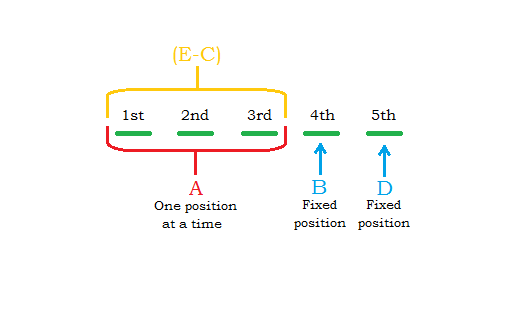Now, let’s answer each question one by one after making diagrammed sketch of the information given in question.

#### 1: (d)

The answer of first question is (d) because we can see that only D is the person that got the 5th rank.

#### 2: (e)

As the question makes further restriction to A, that gives specific place of A. Because the position of A becomes on the place that has next adjacent place of B, who is at 4th rank, therefore the rank of A is 3rd here according to the information of question. So we should change the original diagram according to the information of this question and solve for the first position, as shown below: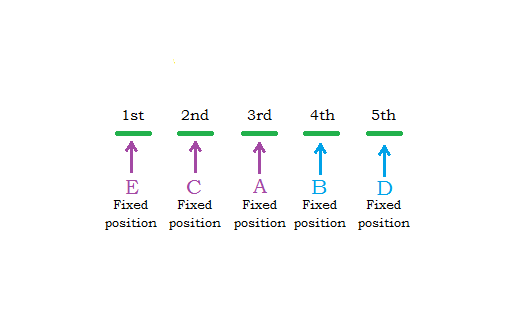#### 3: (c)

To answer this type of question, always start from the original drawing and place the persons to the ranks that are fixed. So we should place B at 4th and D at 5th rank respectively that are fixed. Now as there are 3 persons remaining with three ranks such that E & C will place together, i.e., A can’t be placed between E and C. Therefore only two arrangements are possible. To solve it we need to suppose (E-C) as one pair that can’t be separated or interchanged (i.e. can’t be placed as (C-E), as C will have rank after E. These two possible arrangements are shown below: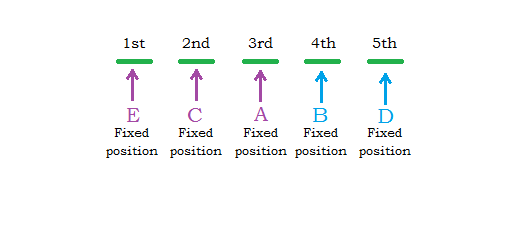Except these two arrangements/order of ranking, any arrangement would be incorrect. So only choice C cannot be the possible arrangement of ranking from Top to Bottom.

#### 4: (c)

Refer to the explanation of Question 3 above. As there are only two possible arrangements of ranking, therefore choice c would be the right answer.

This is the time to do a practice of GAT Analytical Reasoning that will determine whether you have learned GAT Analytical Reasoning Lesson-01 with full dedication? For that purpose, please click on “FREE GAT Analytical Reasoning Practice-01” button below:

Subscribe
Notify of